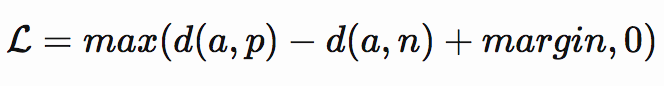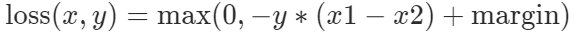# Triplet loss in Pytorch

The equation of triplet loss is:I am trying to implement in this way:

``````type or paste coclass TripletLoss(nn.Module):
"""
Triplet loss
Takes embeddings of an anchor sample, a positive sample and a negative sample
"""

def __init__(self, margin = 1.0):
super(TripletLoss, self).__init__()
self.margin = margin

def forward(self, anchor, positive, negative, size_average=True):
distance_positive = (anchor - positive).pow(2).sum(1)  # .pow(.5)
distance_negative = (anchor - negative).pow(2).sum(1)  # .pow(.5)
losses = F.relu(distance_positive - distance_negative + self.margin)
return losses.mean() if size_average else losses.sum()
de here
``````

Another implementation is like that:

``````class TripletLoss(nn.Module):
"""Triplet loss with hard positive/negative mining.
Reference:
Hermans et al. In Defense of the Triplet Loss for Person Re-Identification. arXiv:1703.07737.
Code imported from https://github.com/Cysu/open-reid/blob/master/reid/loss/triplet.py.
Args:
margin (float): margin for triplet.
"""

def __init__(self, margin=0.3, mutual_flag=False):
super(TripletLoss, self).__init__()
self.margin = margin
self.ranking_loss = nn.MarginRankingLoss(margin=margin)
self.mutual = mutual_flag

def forward(self, inputs, targets):
"""
Args:
inputs: feature matrix with shape (batch_size, feat_dim)
targets: ground truth labels with shape (num_classes)
"""
n = inputs.size(0)
# inputs = 1. * inputs / (torch.norm(inputs, 2, dim=-1, keepdim=True).expand_as(inputs) + 1e-12)
# Compute pairwise distance, replace by the official when merged
dist = torch.pow(inputs, 2).sum(dim=1, keepdim=True).expand(n, n)
dist = dist + dist.t()
dist = dist.clamp(min=1e-12).sqrt()  # for numerical stability
# For each anchor, find the hardest positive and negative
dist_ap, dist_an = [], []
for i in range(n):
dist_ap = torch.cat(dist_ap)
dist_an = torch.cat(dist_an)
# Compute ranking hinge loss
y = torch.ones_like(dist_an)
loss = self.ranking_loss(dist_an, dist_ap, y)
if self.mutual:
return loss, dist
return loss
``````

I understand the first way easily but I am little bit confused in the second way. Can you explain a how can I modify the second one. For an example I need to get another pair consisiting of two negative images. AHow can I get in second way?

1 Like

I have the same question.
Any suggestion?

From Here:
The Margin Ranking Loss measures the loss given inputs x1, x2 , and a label tensor y with values (1 or -1). If y == 1 then it assumed the first input should be ranked higher than the second input, and vice-versa for y == -1.There is a 3rd way which IMHO is the default way of doing it and that is :

``````def triple_loss(a, p, n, margin=0.2) :
d = nn.PairwiseDistance(p=2)
distance = d(a, p) - d(a, n) + margin
loss = torch.mean(torch.max(distance, torch.zeros_like(distance)))
return loss
``````
4 Likes